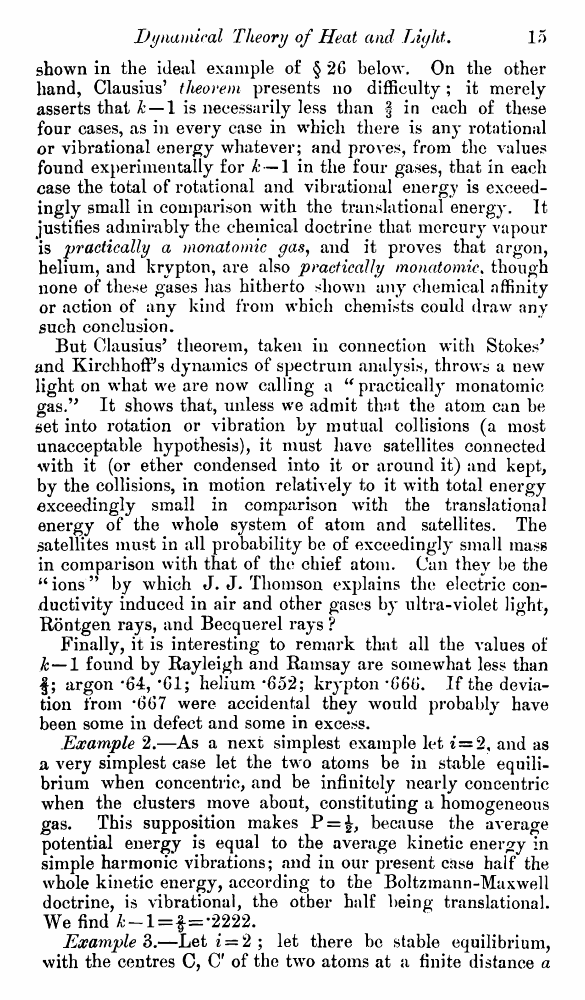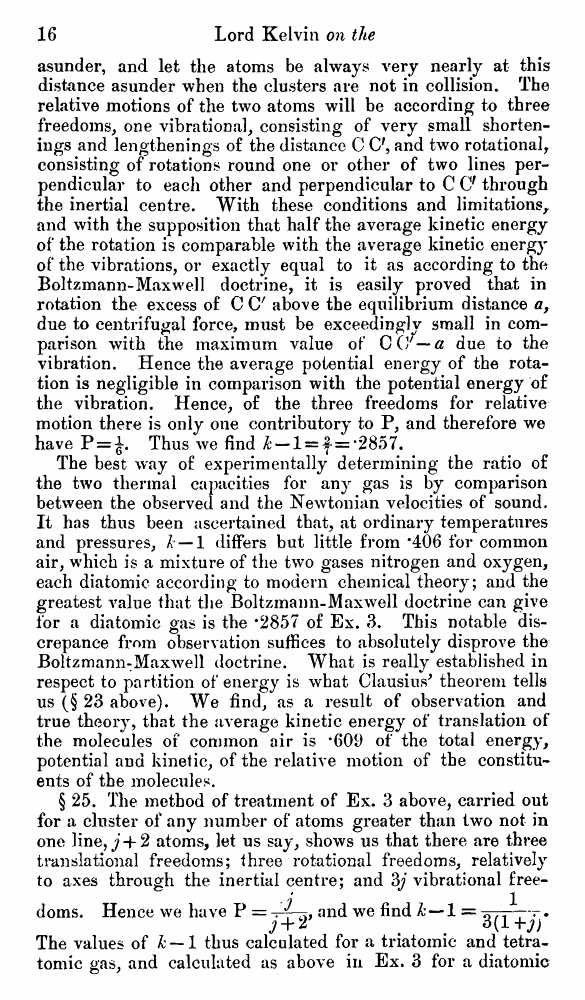# Lord Kelvin. Nineteenth-Century Clouds over the Dynamical Theory of Heat and Light. // Phil. Mag. S. 6. Vol. 2. No. 7. July 1901.

В начало   Другие форматы   <<<     Страница 15   >>>1  2  3  4  5  6  7  8  9  10  11  12  13  14  15 16  17  18  19  20  21  22  23  24  25  26  27  28  29  30  31  32  33  34  35  36  37  38  39  40 shown in the ideal example of § 26 below. On the other hand, Clausius’ theorem presents no difficulty; it merely asserts that k — 1 is necessarily less than § in each of these four cases, as in every case in which there is any rotational or vibrational energy whatever; and proves, from the values found experimentally for k — 1 in the four gases, that in each case the total of rotational and vibrational energy is exceedingly small in comparison with the translational energy. It justifies admirably the chemical doctrine that mercury vapour is practically a monatomic gas, and it proves that argon, helium, and krypton, are also practically monatomic. though none of these gases has hitherto shown any chemical affinity or action of any kind from which chemists could draw any such conclusion. But Clausius’ theorem, taken in connection with Stokes' and KirchhofFs dynamics of spectrum analysis, throws a new light on what we are now calling a “ practically monatomic gas.” It shows that, unless we admit that the atom can be set into rotation or vibration by mutual collisions (a most unacceptable hypothesis), it must have satellites connected with it (or ether condensed into it or around it) and kept, by the collisions, in motion relatively to it with total energy exceedingly small in comparison with the translational energy of the whole system of atom and satellites. The satellites must in all probability be of exceedingly small mass in comparison with that of the chief atom. Can they be the “ions” by which J. J. Thomson explains the electric conductivity induced in air and other gases by ultra-violet light, Rontgen rays, and Becquerel rays ? Finally, it is interesting to remark that all the values of k — 1 found by Rayleigh and Ramsay are somewhat less than f; argon ‘64, *61; helium *652; krypton *666. If the deviation from #667 were accidental they would probably have been some in defect and some in excess. Example 2.—As a next simplest example let 2, and as a very simplest case let the two atoms be in stable equilibrium when concentric, and be infinitely nearly concentric when the clusters move about, constituting a homogeneous gas. This supposition makes P = £, because the average potential energy is equal to the average kinetic energy in simple harmonic vibrations; and in our present case half the whole kinetic energy, according to the Boltzmann-Maxwell doctrine, is vibrational, the other half being translational. We find —l = f=-2222. Example 3.—Let i = 2 ; let there be stable equilibrium, with the centres C, C' of the two atoms at a finite distance a asunder, and let the atoms be always very nearly at this distance asunder when the clusters are not in collision. The relative motions of the two atoms will be according to three freedoms, one vibrational, consisting of very small shortenings and lengthenings of the distance C C', and two rotational, consisting of rotations round one or other of two lines perpendicular to each other and perpendicular to C O through the inertial centre. With these conditions and limitations, and with the supposition that half the average kinetic energy of the rotation is comparable with the average kinetic energy of the vibrations, or exactly equal to it as according to the Boltzmann-Maxwell doctrine, it is easily proved that in rotation the excess of C C' above the equilibrium distance a, due to centrifugal force, must be exceedingly small in comparison with the maximum value of CO7 — a due to the vibration. Hence the average potential energy of the rotation is negligible in comparison with the potential energy of the vibration. Hence, of the three freedoms for relative motion there is only one contributory to P, and therefore we have P = ^. Thus we find & —l = |- = *2857. The best way of experimentally determining the ratio of the two thermal capacities for any gas is by comparison between the observed and the Newtonian velocities of sound. It has thus been ascertained that, at ordinary temperatures and pressures, h — 1 differs but little from *406 for common air, which is a mixture of the two gases nitrogen and oxygen, each diatomic according to modern chemical theory; and the greatest value that the Boltzmann-Maxwell doctrine can give for a diatomic gas is the *2857 of Ex. 3. This notable discrepance from observation suffices to absolutely disprove the Boltzmann:Maxwell doctrine. What is really established in respect to partition of energy is what Clausius' theorem tells us (§ 23 above). We find, as a result of observation and true theory, that the average kinetic energy of translation of the molecules of common air is *609 of the total energy, potential and kinetic, of the relative motion of the constituents of the molecules. § 25. The method of treatment of Ex. 3 above, carried out for a cluster of any number of atoms greater than two not in one line, j-1-2 atoms, let us say, shows us that there are three translational freedoms; three rotational freedoms, relatively to axes through the inertial centre; and 3j vibrational free- ? 1 doms. Hence we have P = —and we find & — 1 = 0.---r• 3 + 1 . . 3(1 +j) The values of k — 1 thus calculated for a triatomic and tetra-tomic gas, and calculated as above in Ex. 3 for a diatomic